# Class 10 Electricity Notes

Electricity Notes

## Charge(Q):-

Electric charge is the basic physical property of matter that causes it to experience a force when kept in an electric or magnetic field.

• It can be positive or negative.
• S.I. unit – Coulomb (C)

## Quantisation of Charge:-

Every charge is an integral multiple of a basic charge i.e., charge on an electron(e).

• Q=ne
• Number of electrons(n) = Q/e
• Charge on an electron (1e) =1.6 x 10-19 C

## Electric Circuit:-

A continuous and closed path of an electric current is called an electric circuit.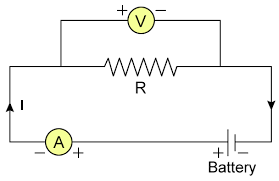## Electric current(I):-

The rate of flow of electric charge is called electric current.

• I = Q/t
• S.I. unit:- Ampere(A)
• Conventionally, the direction of flow of electric current is opposite to the direction of flow of electrons
• The amount of electric current in a circuit is measured by an instrument called ammeter.
• An ammeter is always connected in series in a circuit.
• 1 mA = 10-3 A & 1 µA = 10-6 A

1 Ampere:- The electric current flowing through a circuit is said to be 1 ampere when 1 coulomb of charge flows through it per second.

## Electric Potential:-

The work done to move a unit charge from infinity to a point is known as electric potential of that point.

## Electric Potential Difference:-

The work done to move a unit charge from one point to another point is called potential difference between the two points.

• V = W/Q
• S.I. unit:- Volt(V)
• Potential difference is measured by an instrument called voltmeter.
• Voltmeter is always connected in parallel across the points between which the potential difference is to be measured.

1 Volt:- The potential difference across the two points of a conductor is said to be 1 volt when 1 joule of work is done to move a charge of 1 coulomb from one point to the other.

## Ohm’s Law:-

At constant temperature, the potential difference across the ends of a conductor is directly proportional to the amount of current flowing through it.

Mathematically,
V ∝ I
V = IR where, R is constant of proportionality & is known as resistance.

#### Circuit diagram and V-I graph for Ohm’s law:-

.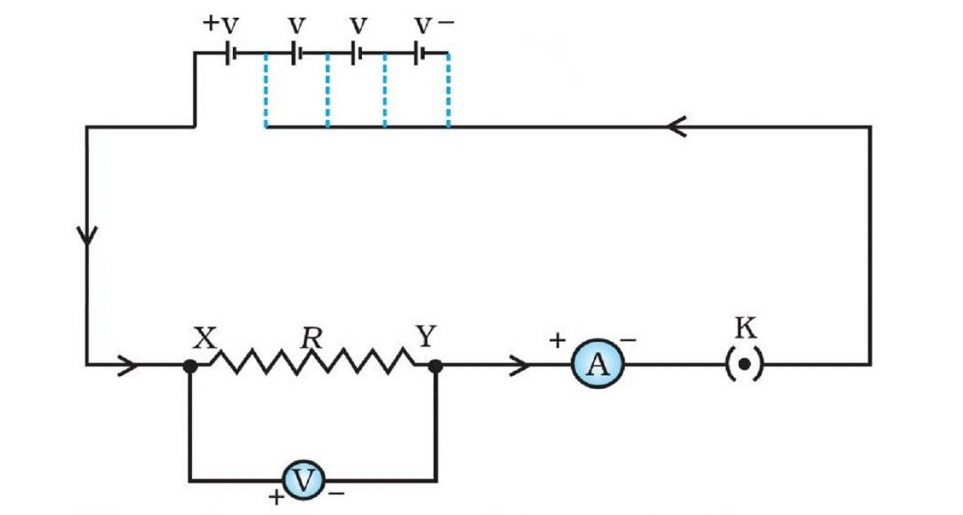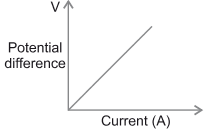## Resistance:-

The property of a conductor to oppose the flow of charges through it is called resistance.

• R = V/I
• S.I. unit:- Ohm (Ω)

1 Ohm:- The resistance of a conductor is said to be 1 Ω if the potential difference across the ends of a conductor is 1 V and the current flowing through it is 1 A.

Resistor:- A conductor having some appreciable resistance

Variable Resistance/Rheostat:- A component used to regulate current without changing the voltage source

## Factors on which resistance of a conductor depends are:-

• its length (l)
• its cross-section area (A)
• nature of its material

Mathematically,
R ∝ l ——–(i)
R ∝ 1/A ——(ii)

On combining (i) & (ii):-
R = ρl / A where, ρ(rho) is constant of proportionality & is known as resistivity

#### Resistivity/Specific Resistance(ρ):-

The resistance of the conductor of unit length & unit area of cross section is called its resistivity.

• ρ =RA/l
• S.I. unit :- ohm meter (Ω m)

On increasing the temperature, both resistance and resistivity of a conductor increases.

## Resistors in Series:-

When two or more resistors are connected end to end to each other, then they are said to be connected in series.

In series combination,

• current flowing is same through each resistor.
• the potential difference across each resistor is different.

.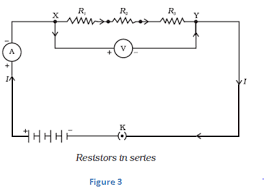Consider current ‘I’ is flowing through the circuit & the potential difference across resistors R1, R2 & R3 be V1, V2, V3 respectively. Let the total potential difference be ‘V’ & equivalent resistance be ‘Rs‘.

On applying Ohm’s law,
V = IRs ; V1 = IR1; V2 = IR2 ; V3 = IR3

Now, V = V1 + V2 + V3
or, IRs = IR1 + IR2 + IR3
or, Rs = R1 + R2 + R3

## Resistors In Parallel:-

When two or more resistors are connected together between two points then they are said to be connected in parallel.

In parallel combination,

• The potential difference is same across each resistor.
• The current flowing through each resistor is different.

.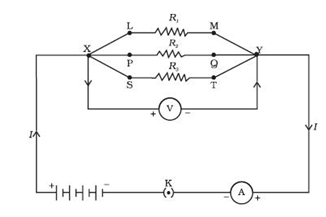Consider potential difference across the resistors be V & the current flowing through resistors R1, R2 & R3 be I1, I2 & I3 respectively. Let the total current flowing through the circuit be I & equivalent resistance be Rp.

By Ohm’s law,
I = V/Rp ; I1 = V/R1 ; I2 = V/R2 ; I3 = V/R3

Now, I = I1 + I2 + I3
or , V/Rp = V/R1 + V/R2 + V/R3
or , 1/Rp = 1/R1 + 1/R2 + 1/R3

## Advantages of parallel combination over series combination:-

• In series combination, current remains same throughout the circuit while in parallel combination, current is different through each device and different components require different currents to operate properly.
• If one device fails in series circuit then none of the devices works while it will not happen in parallel circuit.
• The total resistance in a parallel circuit is decreased while the total resistance in series circuit is increased.

## Electric Power:-

The amount of electrical energy consumed per unit time in an electric circuit is known as electric power.

P = W/t
Or, P = VQ/t
Or, P = VI = I2R = V2/R

• S.I. unit of power:- Watt(W)

1 Watt:- Electric power of an appliance is said to be 1 W if 1 A of current flows through it and the potential difference across its ends is 1 V.

## Heating Effect of Electric Current:-

W = VQ =VIt

or H = VIt = I2Rt

#### Joule’s Law of Heating:-

According to Joule’s law of heating, heat produced in a resistor is directly proportional to

• square of current (H ∝ I2)
• resistance (H ∝ R)
• time for which current flows (H ∝ t)

H = I2Rt

• S.I. unit of energy:- Joule(J)
• Commercial unit of energy:- kilowatt hour(kW h)

#### Practical Applications of Heating Effect of Electric Current:-

Appliances based on heating effect of electric current are electric bulb, electric iron, electric toaster, electric oven, electric kettle, electric heater, fuse etc.

• Tungsten is used as the filament of the electric bulb.
• The bulbs are filled with chemically inactive gases like nitrogen and argon to prolong the life of the filament.

## Conversion of Commercial Unit of Energy to S.I. Unit:-

1 kW h = 1000 W x 3600 s = 3.6 x 106 Ws = 3.6 x 106 J

1 unit = 1 kW h = 3.6 x 106 J• ##### Saurabh singh

Thank you sir for the wonderful notes

68
4
• ##### Vikash Kumar

This notes cleared my doubts. Thank you sir.

67
6
• ##### Vinit Anand

What is the direction of electric current?

71
5
• ##### Kajal

Opposite to the direction of flow of electrons.

67
5
• ##### BLACK1SEVEN

Positive to negative